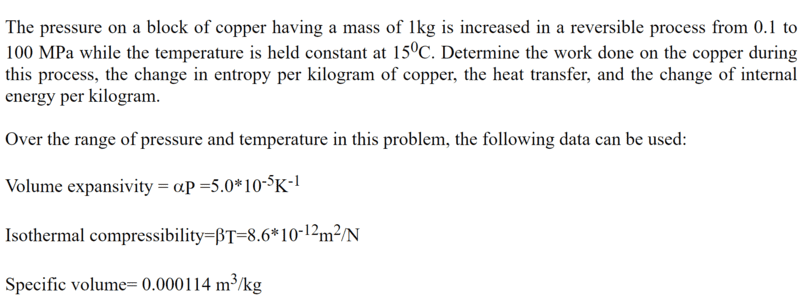Homework StatementQuestion
If changed isothermal compression process to adiabatic compression process. find the final temperature of process.

Homework Equations

## \alpha = \frac{1}{v} (\frac{∂v}{∂T})_{P} ## expansivity
## \beta = -\frac{1}{v} (\frac{∂v}{∂P})_{T} ## compressibility
##P_1^{1-γ}T_1^{γ}=P_2^{1-γ}T_2^{γ}##

##P_1 = 0.1 Mpa##
##P_2 = 100 Mpa##
##T_1 =15+273.15 K##

##γ= \frac{C_p}{C_v}##

The Attempt at a Solution

I don't know γ . So I must find the γ value.
##Tds = \frac{\beta C_v}{\alpha} dP+\frac{C_p}{\alpha v} d##
In adiabatic process ds = 0 So.
## 0= \frac{\beta C_v}{\alpha} dP_s+\frac{C_p}{\alpha v} dv_s##
## -\frac{\beta C_v}{\alpha} dP_s = \frac{C_p}{\alpha v} dv_s##
## -\frac{\beta \alpha v}{\alpha} dP_s = \frac{C_p}{C_v} dv_s##
So
## γ dv_s =- \beta v dP_s ##
## γ =-\beta v (\frac{dP}{dv})_s ##
I don't have a idea to find γ value . Please help me to find final temperature in a Question.

Attachments

Chestermiller
Mentor
You are trying to do the adiabatic reversible compression, correct?

What is the general equation for dS in terms of dT and dP?

•Another
You are trying to do the adiabatic reversible compression, correct?

Yes. sir

What is the general equation for dS in terms of dT and dP?

let s = f(T,P)
##ds = (\frac{∂s}{∂T})_PdT + (\frac{∂s}{∂P})_TdP##

Chestermiller
Mentor
Yes. sir
let s = f(T,P)
##ds = (\frac{∂s}{∂T})_PdT + (\frac{∂s}{∂P})_TdP##
In terms of ##C_P##, what is ##\frac{∂s}{∂T})_P##?
In terms of ##\left(\frac{\partial V}{\partial T}\right)_P##, what is ##(\frac{∂s}{∂P})_T##?

•Another
In terms of ##C_P##, what is ##\frac{∂s}{∂T})_P##?
In terms of ##\left(\frac{\partial V}{\partial T}\right)_P##, what is ##(\frac{∂s}{∂P})_T##?

let ##s = f(P,T)## , ## u = f(P,Y) ## and ## Tds = du + pdv ##

##ds = (\frac{∂s}{∂P})_T dP + (\frac{∂s}{∂T})_P dT##
##du = (\frac{∂u}{∂P})_T dP + (\frac{∂u}{∂T})_P dT##
## Tds = (\frac{∂u}{∂P})_T dP + (\frac{∂u}{∂T})_P dT + Pdv ##
##dp = 0##
## Tds = (\frac{∂u}{∂T})_P dT + Pdv ##
## T(\frac{∂s}{∂T})_P = (\frac{∂u}{∂T})_P + P (\frac{∂v}{∂T})_P ##
## (\frac{∂s}{∂T})_P = \frac{C_P}{T}##

In maxwell relation
##(\frac{∂s}{∂P})_T = - \left(\frac{\partial V}{\partial T}\right)_P##

Chestermiller
Mentor
let ##s = f(P,T)## , ## u = f(P,Y) ## and ## Tds = du + pdv ##

##ds = (\frac{∂s}{∂P})_T dP + (\frac{∂s}{∂T})_P dT##
##du = (\frac{∂u}{∂P})_T dP + (\frac{∂u}{∂T})_P dT##
## Tds = (\frac{∂u}{∂P})_T dP + (\frac{∂u}{∂T})_P dT + Pdv ##
##dp = 0##
## Tds = (\frac{∂u}{∂T})_P dT + Pdv ##
## T(\frac{∂s}{∂T})_P = (\frac{∂u}{∂T})_P + P (\frac{∂v}{∂T})_P ##
## (\frac{∂s}{∂T})_P = \frac{C_P}{T}##

In maxwell relation
##(\frac{∂s}{∂P})_T = - \left(\frac{\partial V}{\partial T}\right)_P##
Good. So, if ds=0, what is dT/dP?

•Another
Good. So, if ds=0, what is dT/dP?
##ds = (\frac{∂s}{∂P})_T dP + (\frac{∂s}{∂T})_P dT##
##0 = (\frac{∂s}{∂P})_T dP + (\frac{∂s}{∂T})_P dT##
## - (\frac{∂s}{∂P})_T dP = (\frac{∂s}{∂T})_P dT##
## (\frac{∂v}{∂T})_P = (\frac{∂s}{∂T})_P (\frac{∂T}{∂P})_s## and ## (\frac{∂v}{∂T})_P = v \alpha ##
## (\frac{∂T}{∂P})_s = \frac{Tv \alpha}{C_p}##

I am not sure

Chestermiller
Mentor
##ds = (\frac{∂s}{∂P})_T dP + (\frac{∂s}{∂T})_P dT##
##0 = (\frac{∂s}{∂P})_T dP + (\frac{∂s}{∂T})_P dT##
## - (\frac{∂s}{∂P})_T dP = (\frac{∂s}{∂T})_P dT##
## (\frac{∂v}{∂T})_P = (\frac{∂s}{∂T})_P (\frac{∂T}{∂P})_s## and ## (\frac{∂v}{∂T})_P = v \alpha ##
## (\frac{∂T}{∂P})_s = \frac{Tv \alpha}{C_p}##

I am not sure
This is correct. So substitute the numbers in and let's see what you get for the temperature rise (don't forget to use absolute temperature).

•Another
This is correct. So substitute the numbers in and let's see what you get for the temperature rise (don't forget to use absolute temperature).
I'm thankful for that.